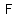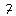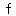# U+2131 SCRIPT CAPITAL F

U+2131 was added to Unicode in version 1.1 (1993). It belongs to the blockLetterlike Symbols in the Basic Multilingual Plane.

This character is a Uppercase Letter and is commonly used, that is, in no specific script. The character is also known as Fourier transform.

The glyph is a Font composition of the glyphs. It has a Neutral East Asian Width. In bidirectional context it acts as Left To Right and is not mirrored. The glyph can, under circumstances, be confused with 29 other glyphs. In text U+2131 behaves as Alphabetic regarding line breaks. It has type Upper for sentence and ALetter for word breaks. The Grapheme Cluster Break is Any.

The Fourier transform decomposes a function of time (e.g., a signal) into the frequencies that make it up, similarly to how a musical chord can be expressed as the amplitude (or loudness) of its constituent notes. The Fourier transform of a function of time itself is a complex-valued function of frequency, whose absolute value represents the amount of that frequency present in the original function, and whose complex argument is the phase offset of the basic sinusoid in that frequency. The Fourier transform is called the frequency domain representation of the original signal. The term Fourier transform refers to both the frequency domain representation and the mathematical operation that associates the frequency domain representation to a function of time. The Fourier transform is not limited to functions of time, but in order to have a unified language, the domain of the original function is commonly referred to as the time domain. For many functions of practical interest one can define an operation that reverses this: the inverse Fourier transformation, also called Fourier synthesis, of a frequency domain representation combines the contributions of all the different frequencies to recover the original function of time.

Linear operations performed in one domain (time or frequency) have corresponding operations in the other domain, which are sometimes easier to perform. The operation of differentiation in the time domain corresponds to multiplication by the frequency, so some differential equations are easier to analyze in the frequency domain. Also, convolution in the time domain corresponds to ordinary multiplication in the frequency domain. Concretely, this means that any linear time-invariant system, such as a filter applied to a signal, can be expressed relatively simply as an operation on frequencies. After performing the desired operations, transformation of the result can be made back to the time domain. Harmonic analysis is the systematic study of the relationship between the frequency and time domains, including the kinds of functions or operations that are "simpler" in one or the other, and has deep connections to almost all areas of modern mathematics.

Functions that are localized in the time domain have Fourier transforms that are spread out across the frequency domain and vice versa, a phenomenon known as the uncertainty principle. The critical case for this principle is the Gaussian function, of substantial importance in probability theory and statistics as well as in the study of physical phenomena exhibiting normal distribution (e.g., diffusion). The Fourier transform of a Gaussian function is another Gaussian function. Joseph Fourier introduced the transform in his study of heat transfer, where Gaussian functions appear as solutions of the heat equation.

The Fourier transform can be formally defined as an improper Riemann integral, making it an integral transform, although this definition is not suitable for many applications requiring a more sophisticated integration theory. For example, many relatively simple applications use the Dirac delta function, which can be treated formally as if it were a function, but the justification requires a mathematically more sophisticated viewpoint. The Fourier transform can also be generalized to functions of several variables on Euclidean space, sending a function of 3-dimensional space to a function of 3-dimensional momentum (or a function of space and time to a function of 4-momentum). This idea makes the spatial Fourier transform very natural in the study of waves, as well as in quantum mechanics, where it is important to be able to represent wave solutions as functions of either space or momentum and sometimes both. In general, functions to which Fourier methods are applicable are complex-valued, and possibly vector-valued. Still further generalization is possible to functions on groups, which, besides the original Fourier transform on ℝ or ℝn (viewed as groups under addition), notably includes the discrete-time Fourier transform (DTFT, group = ℤ), the discrete Fourier transform (DFT, group = ℤ mod N) and the Fourier series or circular Fourier transform (group = S1, the unit circle ≈ closed finite interval with endpoints identified). The latter is routinely employed to handle periodic functions. The Fast Fourier transform (FFT) is an algorithm for computing the DFT.

## Representations

System Representation
8497
UTF-8 E2 84 B1
UTF-16 21 31
UTF-32 00 00 21 31
URL-Quoted %E2%84%B1
HTML-Escape &#x2131;
Wrong windows-1252 Mojibake â±
HTML-Escape &Fouriertrf;
HTML-Escape &Fscr;
alias Fourier transform
LaTeX \mathscr{F}

## Complete Record

Property Value
Age (age) 1.1
Unicode Name (na) SCRIPT CAPITAL F
Unicode 1 Name (na1) SCRIPT F
Block (blk) Letterlike_Symbols
General Category (gc) Uppercase Letter
Script (sc) Common
Bidirectional Category (bc) Left To Right
Combining Class (ccc) Not Reordered
Decomposition Type (dt) Font
Decomposition Mapping (dm)Lowercase (Lower)
Simple Lowercase Mapping (slc)Lowercase Mapping (lc)Uppercase (Upper)
Simple Uppercase Mapping (suc)Uppercase Mapping (uc)Simple Titlecase Mapping (stc)Titlecase Mapping (tc)Case Folding (cf)ASCII Hex Digit (AHex)
Alphabetic (Alpha)
Bidi Control (Bidi_C)
Bidi Mirrored (Bidi_M)
Bidi Paired Bracket (bpb)Bidi Paired Bracket Type (bpt) None
Cased (Cased)
Composition Exclusion (CE)
Case Ignorable (CI)
Full Composition Exclusion (Comp_Ex)
Changes When Casefolded (CWCF)
Changes When Casemapped (CWCM)
Changes When NFKC Casefolded (CWKCF)
Changes When Lowercased (CWL)
Changes When Titlecased (CWT)
Changes When Uppercased (CWU)
Dash (Dash)
Deprecated (Dep)
Default Ignorable Code Point (DI)
Diacritic (Dia)
East Asian Width (ea) Neutral
Extender (Ext)
FC NFKC Closure (FC_NFKC)Grapheme Cluster Break (GCB) Any
Grapheme Base (Gr_Base)
Grapheme Extend (Gr_Ext)
Hex Digit (Hex)
Hangul Syllable Type (hst) Not Applicable
Hyphen (Hyphen)
ID Continue (IDC)
Ideographic (Ideo)
ID Start (IDS)
IDS Binary Operator (IDSB)
IDS Trinary Operator and (IDST)
InMC (InMC)
Indic Positional Category (InPC) NA
Indic Syllabic Category (InSC) Other
ISO 10646 Comment (isc)
Joining Group (jg) No_Joining_Group
Join Control (Join_C)
Jamo Short Name (JSN)
Joining Type (jt) Non Joining
Line Break (lb) Alphabetic
Logical Order Exception (LOE)
Math (Math)
Noncharacter Code Point (NChar)
NFC Quick Check (NFC_QC) Yes
NFD Quick Check (NFD_QC) Yes
NFKC Casefold (NFKC_CF)NFKC Quick Check (NFKC_QC) No
NFKD Quick Check (NFKD_QC) No
Numeric Type (nt) None
Numeric Value (nv) NaN
Other Alphabetic (OAlpha)
Other Default Ignorable Code Point (ODI)
Other Grapheme Extend (OGr_Ext)
Other ID Continue (OIDC)
Other ID Start (OIDS)
Other Lowercase (OLower)
Other Math (OMath)
Other Uppercase (OUpper)
Pattern Syntax (Pat_Syn)
Pattern White Space (Pat_WS)
Quotation Mark (QMark)
Sentence Break (SB) Upper
Simple Case Folding (scf)Script Extension (scx) Common
Soft Dotted (SD)
STerm (STerm)
Terminal Punctuation (Term)
Unified Ideograph (UIdeo)
Variation Selector (VS)
Word Break (WB) ALetter
White Space (WSpace)
XID Continue (XIDC)
XID Start (XIDS)
Expands On NFC (XO_NFC)
Expands On NFD (XO_NFD)
Expands On NFKC (XO_NFKC)
Expands On NFKD (XO_NFKD)